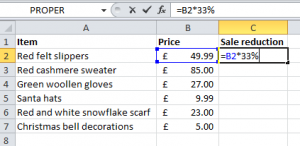# How to calculate percentage reduction using Excel formulas

One of the many things I like about Excel is that I can set up the formula in one cell and copy it to other cells.

If I want to apply a percentage reduction to a figure, I can set it up for the first line of data and then autofill the relevant cells.  With adverts already reminding us to spend for Christmas and plan for the sales, here is a simple example of applying a percentage price reduction in Excel.

Applying the same percentage discount to multiple items
A shop wants to calculate discounts of 33% for all sale items in the January sale, and an Excel sheet can set these up easily.

All the items will have a 33% reduction applied on 1st January 2013.I create a formula using the data in B2 and then copy the formula to the remaining cells in the column

To set up the formula in the first cell, the price in B2 is selected, and then multiplied by 33%.  The formula will appear as =B2*33%.  By clicking enter the discount is applied.  This formula can now be copied down the column.